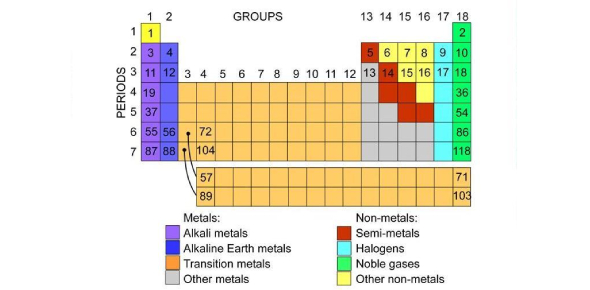# The Periodic Table Quiz: Name The Group Of Elements!

10 Questions | Attempts: 2006
ShareSettingsThe periodic table classifies elements based on shared characteristics. Each group has a specific name, and most of the elements react the same way. Do you have a clear understanding of the different groups and the elements in it? If so, then take up the quiz below and get to test your knowledge. All the best and keep revising!

• 1.
What is the group name of helium?
• A.

Alkali matals

• B.

Halogens

• C.

Alkaline earth metals

• D.

Noble gases

• 2.
What is the name of horizontal rows in the periodic table?
• A.

Groups

• B.

Periods

• C.

Families

• D.

Classes

• 3.
What are the last two types of elements to be added to the Periodic Table?
• A.

Halogens and noble gases

• B.

Alkali metals and halogens

• C.

Alkaline earth metals and alkali metals

• D.

Noble gases and alkaline earth metals

• 4.
Which feature on the Periodic Table is used to identify an element?
• A.

Atomic mass

• B.

Groups

• C.

Atomic number

• D.

Periods

• 5.
Most of the elements on the periodic table are metals?
• A.

True

• B.

False

• 6.
The atomic number of an element is same as the number of?
• A.

Neutrons

• B.

Protons

• C.

Protons + neutrons

• D.

Protons + electrons

• 7.
What is the symbol for sodium?
• A.

K

• B.

Si

• C.

Na

• D.

Sn

• 8.
Sulfur is non-metal.
• A.

True

• B.

False

• 9.
The sodium atom contains 11 electrons, 11 protons and 12 neutrons. What is the mass number of sodium?
• A.

11

• B.

22

• C.

23

• D.

34

• 10.
Which group contains elements that are the most reactive?
• A.

Halogens

• B.

Alkali metals

• C.

Noble gases

• D.

Alkaline earth metals

## Related TopicsBack to top
×

Wait!
Here's an interesting quiz for you.